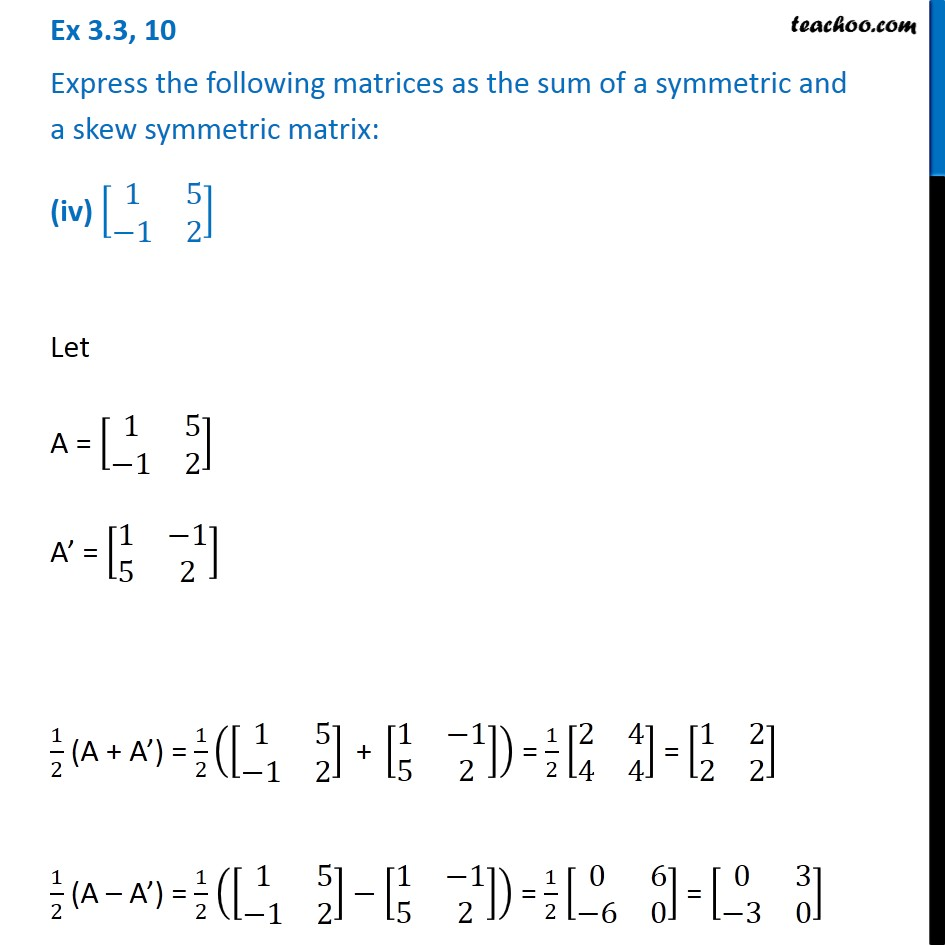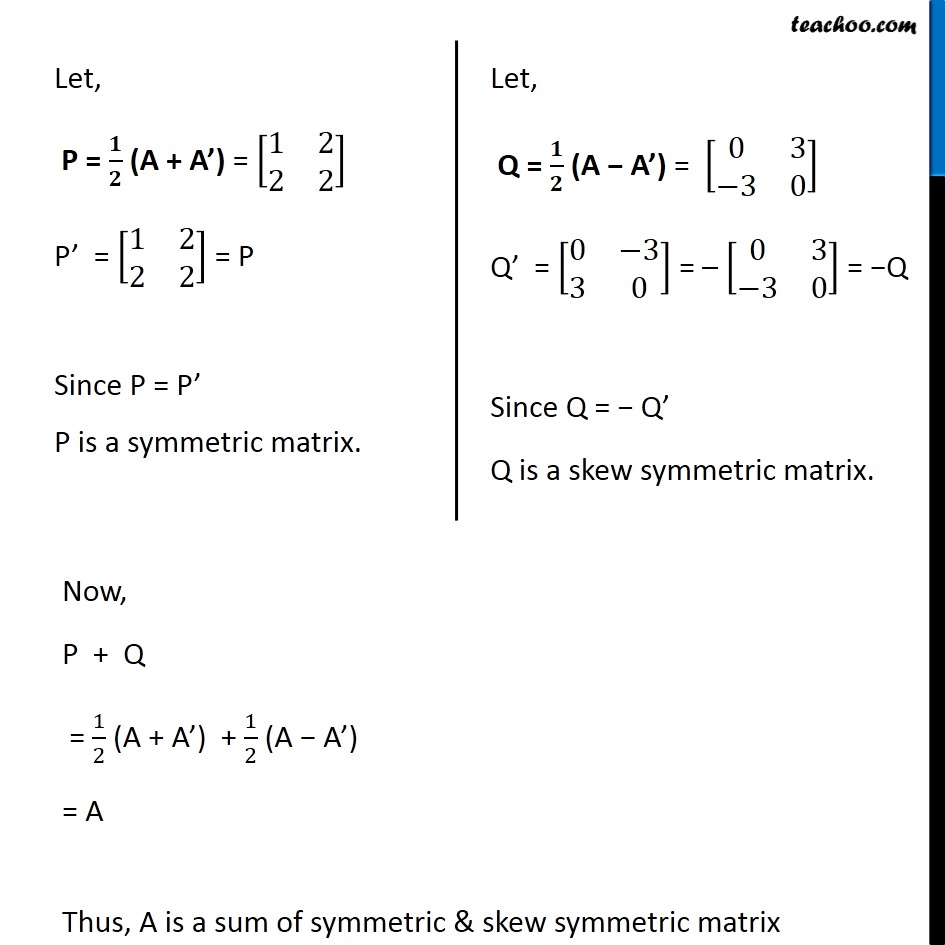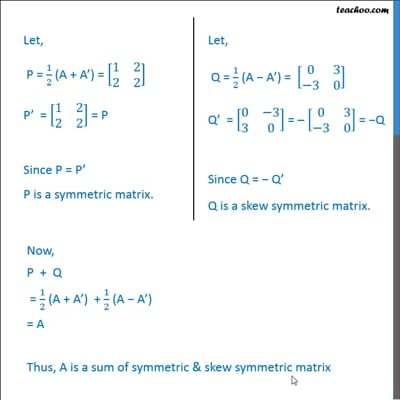Ex 3.3

Chapter 3 Class 12 Matrices
Serial order wiseThis video is only available for Teachoo black users

Maths Crash Course - Live lectures + all videos + Real time Doubt solving!

### Transcript

Ex 3.3, 10 Express the following matrices as the sum of a symmetric and a skew symmetric matrix: (iv) [■8(1&[email protected]−1&2)] Let A = [■8(1&[email protected]−1&2)] A’ = [■8(1&−[email protected]&2)] 1/2 (A + A’) = 1/2 ([■8(1&[email protected]−1&2)]" + " [■8(1&−[email protected]&2)]) = 1/2 [■8(2&[email protected]&4)] = [■8(1&[email protected]&2)] 1/2 (A – A’) = 1/2 ([■8(1&[email protected]−1&2)]−[■8(1&−[email protected]&2)]) = 1/2 [■8(0&[email protected]−6&0)] = [■8(0&[email protected]−3&0)] Let, P = 𝟏/𝟐 (A + A’) = [■8(1&[email protected]&2)] P’ = [■8(1&[email protected]&2)] = P Since P = P’ P is a symmetric matrix. Let, Q = 𝟏/𝟐 (A − A’) = [■8(0&[email protected]−3&0)] Q’ = [■8(0&−[email protected]&0)] = – [■8(0&[email protected]−3&0)] = −Q Since Q = − Q’ Q is a skew symmetric matrix. Now, P + Q = 1/2 (A + A’) + 1/2 (A − A’) = A Thus, A is a sum of symmetric & skew symmetric matrix